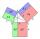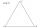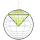# Pythagorean theorem + triangle - math problems

#### Number of problems found: 683

• LawsFrom which law follows directly the validity of Pythagoras' theorem in the right triangle? ...
• Right triangleIt is given a right triangle angle alpha of 90 degrees beta angle of 55 degrees c = 10 cm use Pythagorean theorem to calculate sides a and bFind the radius of the circle using the Pythagorean theorem where a=9, b=r, c= 6+r
• TriangleCan an equilateral triangle have a right angle?
• Euclid2In right triangle ABC with right angle at C is given side a=29 and height v=17. Calculate the perimeter of the triangle.
• Right triangle trigonometricsCalculate the size of the remaining sides and angles of a right triangle ABC if it is given: b = 10 cm; c = 20 cm; angle alpha = 60° and the angle beta = 30° (use the Pythagorean theorem and functions sine, cosine, tangent, cotangent)
• Triangle ABCTriangle ABC has side lengths m-1, m-2, m-3. What has to be m to be triangle a) rectangular b) acute-angled?
• Vertex pointsGiven the following points of a triangle: P(-12,6), Q(4,0), R(-8,-6). Graph the triangle. Find the triangle area.
• Height 2Calculate the height of the equilateral triangle with side 27.
• Area of a rectangleCalculate a rectangle area with a diagonal of u = 12.5cm and a width of b = 3.5cm. Use the Pythagorean theorem.
• Equilateral triangle v2Equilateral triangle has a perimeter 36 dm. What is its area?
• TriangleCalculate the triangle sides if its area S = 630 and the second cathetus is shorter by 17.
• Equilateral triangleFind the area of an equilateral triangle with a side of 15 cm.
• Height UTHow long is height in the equilateral triangle with a side b = 43?
• Equilateral triangleCalculate the area of an equilateral triangle with circumference 72cm.
• Equilateral triangleThe equilateral triangle has a 23 cm long side. Calculate its content area.
• Acceleration 2if a car traveling at a velocity of 80 m/s/south accelerated to a velocity of 100 m/s east in 5 seconds, what is the cars acceleration? using Pythagorean theorem
• Equilateral triangleCalculate the side of an equilateral triangle, if its area is 892 mm2.
• Is right triangleDecide if the triangle XYZ is rectangular: x = 4 m, y = 6 m, z = 4 m
• The rotatingThe rotating cone has a height of 0.9 m and the diameter of the base is 7.2 dm. Calculate the surface of the cone. (Hint: use Pythagorean theorem for a side of cone)

Do you have an interesting mathematical word problem that you can't solve it? Submit a math problem, and we can try to solve it.

We will send a solution to your e-mail address. Solved examples are also published here. Please enter the e-mail correctly and check whether you don't have a full mailbox.

Please do not submit problems from current active competitions such as Mathematical Olympiad, correspondence seminars etc...

See also our trigonometric triangle calculator. Pythagorean theorem is the base for the right triangle calculator. Pythagorean theorem - math problems. Triangle Problems.